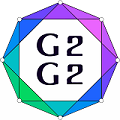#Graphs and Groups, Geometries and GAP (G2G2) Summer School - External Satellite Conference of 8ECM

27 June 2021 to 3 July 2021
Rogla, Slovenia
UTC timezone

## On the Weisfeiler-Leman dimension of some classes of graphs

Not scheduled
20m
Rogla, Slovenia

#### Rogla, Slovenia

Hotel Planja, Rogla 1, 3214 Zreče, Slovenia
Oral presentation

### Speaker

Grigory Ryabov (Sobolev Institute of Mathematics and Novosibirsk State University)

### Description

The Weisfeiler-Leman algorithm (WL-algorithm) is a fundamental algorithm used as a subroutine in graph isomorphism testing. The classical WL-algorithm  constructs for a given graph the coherent closure of its edge set in polynomial time. For every positive integer $k$ there is a $k$-dimensional version of the WL-algorithm which deals with $k$-tuples of vertices (see Section 4.6  for details). In this terms the classical WL-algorithm is $2$-dimensional.

Following Grohe (Definition 18.4.2 in ), we define the WL-dimension $dim_{WL}(\mathcal{K})$ of a class $\mathcal{K}$ of graphs to be the minimal integer $m$ such that the $m$-dimensional WL-algorithm distinguishes each graph $\Gamma\in \mathcal{K}$ from all graphs not isomorphic to $\Gamma$. If $dim_{WL}(\mathcal{K})=m$ then the isomorphism of two $n$-vertex graphs from $\mathcal{K}$ can be checked in time $n^{O(m)}$. The WL-dimension is bounded for many natural graph classes including trees, cographs, interval graphs, planar graphs. In the talk we discuss on the approach to estimate an upper bound of the WL-dimension based on separability property of coherent configurations and present new upper bounds for the WL-dimension of some classes of graphs.

References:

 G. Chen, I. Ponomarenko, Coherent configurations, Central China Normal University Press, Wuhan (2019).

 M. Grohe, Descriptive complexity, canonisation, and definable graph structure theory, Cambridge University Press, Cambridge (2017).

 B. Weisfeiler, A. Leman, Reduction of a graph to a canonical form and an algebra which appears in the process. NTI 2, No. 9 (1968) 12--16.

### Primary authors

Grigory Ryabov (Sobolev Institute of Mathematics and Novosibirsk State University) Prof. Ilia Ponomarenko (St.Petersburg Department of the Steklov Mathematical Institute)